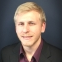# QlikView App Dev

Discussion Board for collaboration related to QlikView App Development.

Announcements
Become an analytics expert with Qlik's new 15 week course: Applied Data Analytics using Qlik Sense. READ MORE
cancel
Showing results for
Did you mean:Contributor III

## Set Analysis Qtr, max(Month)

I am trying to build an expression that shows the sum of the balances for the previous quarter's max month. Here's the variables and function I have:

```previousQuarter = 'Q' & (Right(Quarter, 1) - 1)
maxMonthSelected =max([Deposit Month])
=sum ( {< Quarter = {\$(=previousQuarter)}, [Deposit Month] = {\$(=maxMonthSelected)} >} [Current Balance Amount] )

```

This is returning 0, which isn't correct. The variables themselves are returning what I expect except if Quarter = Q1, which I'll have to fix later. Am I missing something in my set expression? I've tried swapping the variables for the expressions of the variables and the syntax checker doesn't accept it.

Thoughts?

EDIT: Added (working...?) sample at Sunny's request.

Message was edited by: Tyler Tollin

1 Solution

Accepted SolutionsMVP

So, this shows you the right Month to display?

=Max({<Quarter = {\$(=previousQuarter)}>} [Deposit Month])

May you you need Single/Double quotes within dollar sign expansion

=Sum({<Quarter = {"\$(=previousQuarter)"}, [Deposit Month] = {"\$(=maxMonthPrevQtr)"}>} [Current Balance Amount])

8 RepliesMVP

May be try this

previousQuarter = 'Q' & (Right(Quarter, 1) - 1)

maxMonthSelected = Max({<Quarter = {\$(=previousQuarter)}>} [Deposit Month])

=Sum({<Quarter = {\$(=previousQuarter)}, [Deposit Month] = {\$(=maxMonthSelected)}>} [Current Balance Amount] )Contributor III
Author

Thanks for helping, Sunny.

I've either done something wrong, or it isn't working. I created a new variable:

maxMonthPrevQtr =Max( {<Quarter = {\$(=previousQuarter)}>} [Deposit Month])

and inserted it into the original function:

=sum ( {< Quarter = {\$(=previousQuarter)}, [Deposit Month] = {\$(=maxMonthPrevQtr)} >} [Current Balance Amount] )

This is still returning 0. The new variable, if put into a textbox alone, works as expected.MVP

So, this shows you the right Month to display?

=Max({<Quarter = {\$(=previousQuarter)}>} [Deposit Month])

May you you need Single/Double quotes within dollar sign expansion

=Sum({<Quarter = {"\$(=previousQuarter)"}, [Deposit Month] = {"\$(=maxMonthPrevQtr)"}>} [Current Balance Amount])Contributor III
Author

That variable does work as intended, yes. IE: selecting Q2 2017 returns month = 3.

I tried both single and double quotes, no luck.MVP

Would you be able to share a sample to test this out?Contributor III
Author

Sunny,

I added a sample application... which is working as you've described. I'll go back to my actual app and compare and see what's different and if I can fix it.MVP

Sounds goodContributor III
Author

I was able to get this working by putting my month and year fields in Num(). I'm still working on the =if() for when Quarter = Q1 and I need Q4 of previous year data.

Going to mark this as closed; thanks for you help as always, Sunny!